Multiplying Binomials Terms of Use    Contact Person: Donna RobertsDirections: Answer these questions pertaining to polynomials. Check each answer when finished.

1.
Multiply:   (a - 1)(a + 4)
Choose:
 a2 + 5a - 4 a2 + 5a + 4 a2 + 3a - 4 a2 - 3a - 42.
Which choice is equivalent to:   9x2 - 4
Choose:
 (3x - 2)(3x + 2) (3x + 2)2 (3x - 2)2 (x + 1)(9x - 4)3.
Which choice is equivalent to:   (2m - 1)2
Choose:
 4m2 + 2m + 1 4m2 - 4m + 1 4m2 - 2m + 1 4m2 + 14.
Multiply:   (3x + 2y)(x + y)
Choose:
 3x2 + 6xy + 2y2 3x2 + 2y2 3x2 + 5xy + 2y2 3x2 + 3xy + 2y25.
Multiply: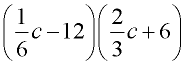Choose: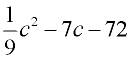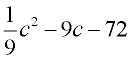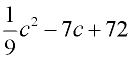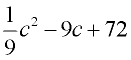6.
The base of a triangle is represented by (x + 4) and its height to that side is represented by (x - 3). Express the area of the triangle.
Choose: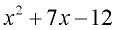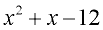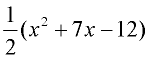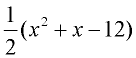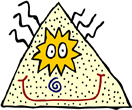7.
Find:   (2x3 + y2)(2x3 - y2)
Choose:
 4x3 - y2 4x3 + y2 4x6 - y4 4x6 + y4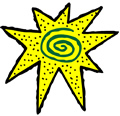8.
Find the values for a, b, and c such that:
(2x + 1)(3x - 4) = ax2 + bx + c

Choose:
 a = 6, b = 5, c = 4 a = 6, b = -5, c = -4 a = 6, b = 0, c = -4 a = 6, b = 11, c = -49.
Which choice is equivalent to:   (a - 3)2 - 9
Choose:
 a2 + 6a a2 - 6 a2 + 6 a2 - 6a10.
Which choice is equivalent to:   (x2 + 3)(x2 + 1)
Choose:
 x2 + 4x + 3 x4 + 3 x4 + 4x2 + 3 x4 + 4x3 + 3x2